# How to use the IFERROR Function in Excel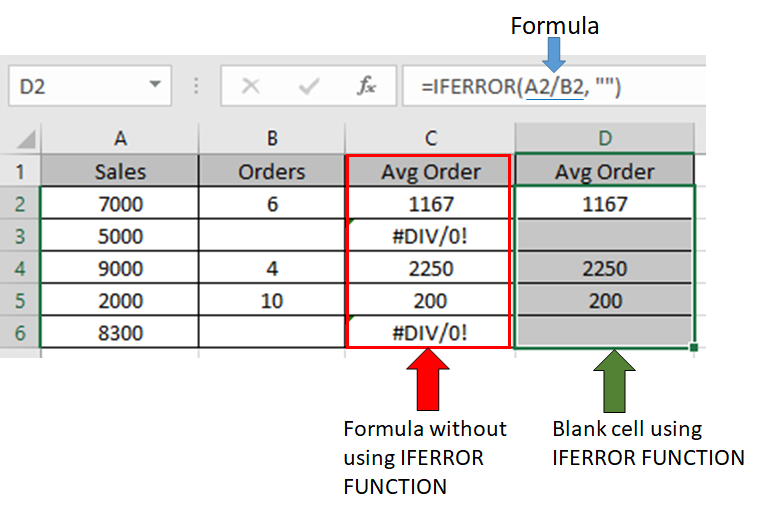In this article, we will learn How to use the IFERROR Function in Excel.

how to use IFERROR function to ignore the #N/A error from a formula

Formula return value or error. Sometimes we need to know the type of error occurring. But sometimes we don't need. In return we just need an empty cell or some text like No value found or Not applicable or Don't know (user defined text). For this Excel provide us with an option or using a function named IFERROR function. Just use the formula inside this function and return whatever value you need to replace with any error.

IFERROR Function in Excel

IFERROR function runs the logic_test in Excel. It catches error in a formula and returns an alternative result or formula when an error is detected. #N/A, #VALUE!, #REF!, #DIV/0!, #NUM!, #NAME?, and #NULL!  These errors are checked under IFERROR.

Syntax:

 =IFERROR (formula, value_if_error)

formula : use the formula inside the IFERROR function

value_if_error : text like "No value found" or "Not applicable" or "Don't know" are some user defined texts (use quotation marks)

Example :

All of these might be confusing to understand. Let's understand how to use the function using an example. Here we have some sales values and orders. We need to use a simple formula in Excel which returns the value and ignore errors wherever occured.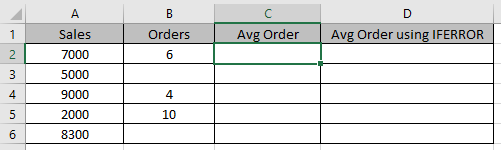Use the formula in the C2 cell.

 =A2/B2

Copy the formula in other cells, select the cells taking the first cell where the formula is already applied, use shortcut key Ctrl + D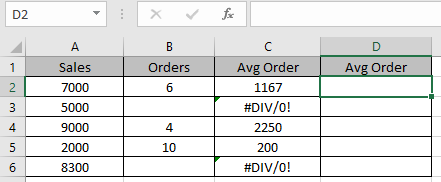As you can see it generates division error here.

Use the IFERROR formula

 =IFERROR(A2/B2, “”)

This formula returns blank cell if error is generated in the division operator else it returns the value after division.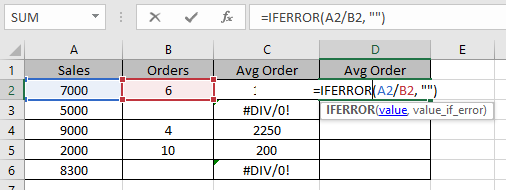Press Enter and Copy the formula in other cells, select the cells taking the first cell where the formula is already applied, use shortcut key Ctrl + D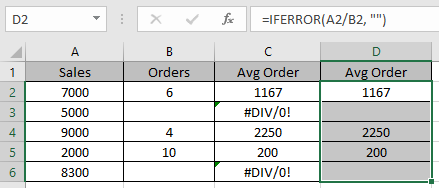That’s the difference it makes.

IFERROR function using other functions like VLOOKUP

Let’s take an example and understand how we can ignore VLOOKUP #N/A Error in Excel.

Here we have two sheets, we have to get the BIRTHDAY from SHEET 1 table to SHEET 2 Table using the functions.

SHEET 1: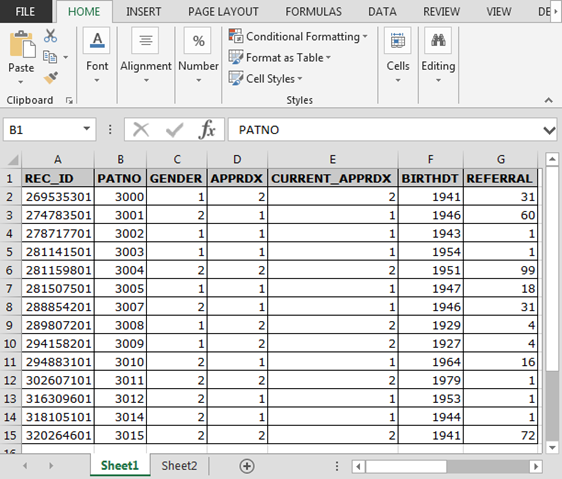SHEET 2: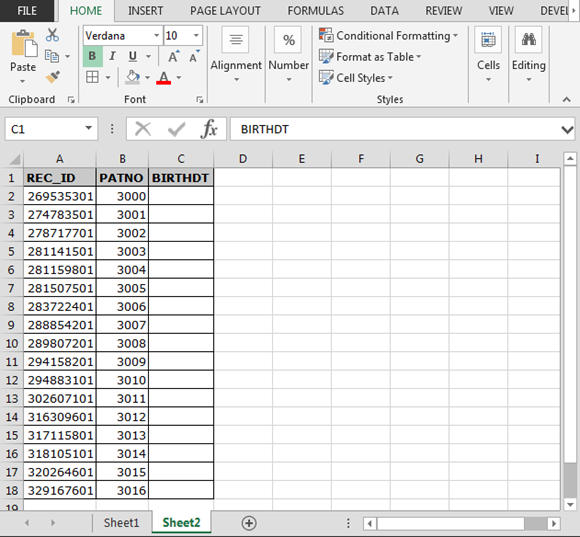Write the IFERROR formula in C2 cell of SHEET 2.

 =IFERROR(VLOOKUP(A2,Sheet1!A:F,6,FALSE),"NotFound")

How it works:

VLOOKUP(A2,Sheet1!A:F,6,FALSE) : The VLOOKUP function looks for the 6th column of the SHEET 1 matching  REC_ID in SHEET 1 from  SHEET 2.

IFERROR catches the error in the VLOOKUP formula and returns “Not Found” (user defined)

Copy the formula in other cells, select the cells taking the first cell where the formula is already applied, use shortcut key Ctrl + D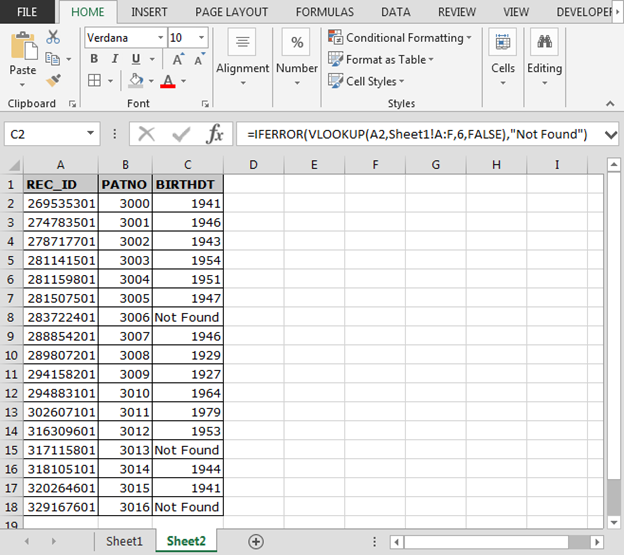As you can see in the above image that formula is working fine. And the user can work without getting irritated by #NA error.

Here are all the observational notes using the IFERROR function in Excel
Notes :

1. You can return any text or blank value to replace any error occured using any formula
2. Use text using double quotation marks as shown in example.
3. You will not be able to detect the type of error as the function works same for all the errors.

Hope this article about How to use the IFERROR Function in Excel is explanatory. Find more articles on calculating values and related Excel formulas here. If you liked our blogs, share it with your friends on Facebook. And also you can follow us on Twitter and Facebook. We would love to hear from you, do let us know how we can improve, complement or innovate our work and make it better for you. Write to us at info@exceltip.com.

Related Articles :

WHY #VALUE! Error occur And How to Fix It in Excel : Detect the unsupported variable in formula using the #VALUE! Error. Learn how to resolve different #VALUE! Error in Excel.

How to correct a #NUM! ErrorDetect the disallowed number with some functions like SQRT function using the #NUM! Error. Resolve this error using the ISERROR function to get more readable data in Excel.

Why #NAME? occur and How to fix #NAME? Error : Detect the unsupported or unrecognized keyword in formula using the #NAME? Error. Learn how to resolve different #NAME? Error in Excel.

Why #DIV/0! occur and How to fix #DIV/0! Error : Detect the mathematical errors like division by zero in formula using the #DIV/0! Error. Learn how to resolve different #DIV/0! Error in Excel.

Why #N/A occur and How to fix #N/A error : Detect the unavailability of value in dataset with formula using the #N/A Error. Resolve this error using the IFERROR or IFNA functions to get more readable data in Excel.

Why #NULL! occur and How to fix #NULL error : Detect the disallowed format in formula using the #NUM! Error. Learn the correct formula to resolve the #NULL Error in Excel.

Why #REF! occur and How to fix #REF! error : Detect the incorrect reference in formula using the #REF! Error. Learn the correct formula to resolve the #REF! Error in Excel.

Popular Articles :

How to use the IF Function in Excel : The IF statement in Excel checks the condition and returns a specific value if the condition is TRUE or returns another specific value if FALSE.

How to use the VLOOKUP Function in Excel : This is one of the most used and popular functions of excel that is used to lookup value from different ranges and sheets.

How to use the SUMIF Function in Excel : This is another dashboard essential function. This helps you sum up values on specific conditions.

How to use the COUNTIF Function in Excel : Count values with conditions using this amazing function. You don't need to filter your data to count specific values. Countif function is essential to prepare your dashboard.

Terms and Conditions of use

The applications/code on this site are distributed as is and without warranties or liability. In no event shall the owner of the copyrights, or the authors of the applications/code be liable for any loss of profit, any problems or any damage resulting from the use or evaluation of the applications/code.